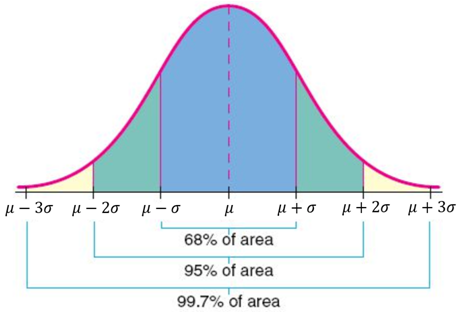Introduction to normal distribution

Introduction to normal distribution

Lessons

Properties of a Normal Distribution
$\cdot$ About 68% of the population are within 1 standard deviation of the mean.
$\cdot$ About 95% of the population are within 2 standard deviations of the mean.
$\cdot$ About 99.7% of the population are within 3 standard deviations of the mean.

.Calculator Commands
$\cdot$ To calculate the normal distribution probability between two data values:
normalcdf (lower bound, upper bound, mean, standard deviation)
- To calculate the area to the left of a data value, replace the lower bound by $-1 \times 10^{99}$
- To calculate the area to the right of a data value, replace the upper bound by $1 \times 10^{99}$
$\cdot$ To calculate a data value, given the area to the left of the data value:
invNorm (area, mean, standard deviation)
• Introduction
Introducing the Normal Distribution
$\cdot$ bell-shaped curve
$\cdot$ Human characteristics such as height, weight, IQ score have frequency graphs that closely approximate a normal distribution.
$\cdot$ 68-95-99.7 Rule

• 1.
The Normal Distribution and the 68-95-99.7 Rule
The weight of chocolate bars produced by a factory is normally distributed with a mean of 225 grams and a standard deviation of 5 grams. Determine the percentage of the chocolate bars that could be expected to weigh

a) between 220 and 230 grams.
b) between 215 and 235 grams.
c) between 210 and 240 grams.
d) between 225 and 230 grams.
e) between 230 and 235 grams.
f) between 210 and 215 grams.
g) between 220 and 240 grams.
h) above 225 grams.
i) above 240 grams.
j) below 220 grams.

• 2.
Using "invNorm" to Solve Normal Distribution Problems
The weight of chocolate bars produced by a factory is normally distributed with a mean of 225 grams and a standard deviation of 5 grams.
a)
The factory has an acceptable minimum weight and all chocolate bars below the minimum weight standard are rejected. If 7% of the chocolate bars are rejected, determine the acceptable minimum weight.

b)
If the heaviest 10% of the chocolate bars are classified as "Supersize Chocolate Bars", what is the weight of the lightest Supersize Chocolate Bar?